# Optimum Drilling Flow Rate Example Calculation

This topic will demonstrate how to determine optimum drilling flow rate for both drilling hydraulic optimization methods (the maximum hydraulic horse power and the maximum impact force). If you don’t know the equations, please read this article first, Determine Optimum Drilling Flow Rate for Basic System – Drilling Hydraulics.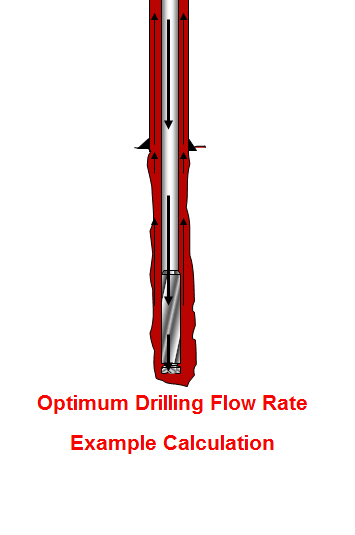The information is listed below:

Hole size = 8.5 inch

Depth In = 2,000 ft

Depth out = 6,000 ft

Mud properties at starting point

Mud weight = 9.2 ppg

PV = 13

YP = 10

Mud properties at TD

Mud weight = 9.5 ppg

PV = 14

YP = 11

Drill pipe = 5”

Drill pipe ID = 4.2”

Drill pipe tool joint = 6.5”

Drill pipe tool joint ID = 3.5

Drill collar = 6-3/4”

Drill collar ID = 3-1/2”

Drill collar length = 800 ft

Coefficient of surface equipment = 5

Pump Capacity with 6” liner, Triplex pump:

Max flow rate = 900 gpm

Max Pressure = 4500 psi

The best optimization point is at the end so this calculation will be based on Drilling Hydraulics Optimized at the End of the Run.

Coefficient for drill pipe and tool joint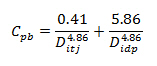Where;

Cpb = coefficient through inside of pipe and tool joint

Ditj = internal diameter of tool joint, inch

Didp = internal diameter of drill pipe, inch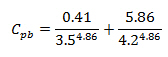Cpb = 0.006243486

Coefficient of Annulus around Drill Pipe and Tool JointWhere:

Cpa = coefficient for the annulus of pipe

Dh = hole diameter, inch

Dp = outside diameter of drill pipe, inch

Dj = outside diameter of tool joint, inch

B = a parameter which takes into account the difference between Q1.86 and Q2. You can just simply select “B” value from the following table.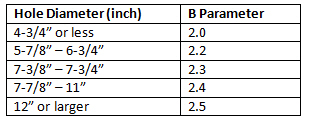Cpa = 0.003082686

Total pressure loss coefficient for drill pipe and tool joint

Cp = Cpb + Cpa

Cp = 0.009326172

Coefficient for drill collar

Inside of drill collar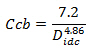Where;

Ccb = coefficient through inside of drill collar

Didc = internal diameter of drill collar, inch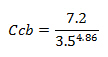Ccb = 0.016337

Annulus of drill collarWhere:

Cca = coefficient for the annulus of drill collar

Dh = hole diameter, inch

Dc = outside diameter of drill collar, inch

B = a parameter which takes into account the difference between Q1.86 and Q2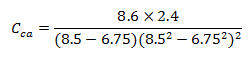Cca = 0.016559829

Total pressure loss coefficient for drill collar

Cc = Ccb + Cca

Cc = 0.032896

Coefficient for surface equipment

Cse = 5

Viscosity Correction Factor

Vf = (15÷9.5)0.14

Vf= 1.055788

Determine optimum flow rate

Maximum Hydraulic Horsepower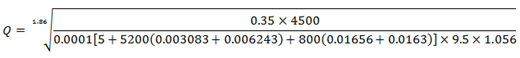Q = 702 gpm

Maximum Impact Force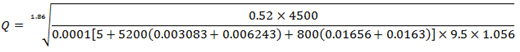Q = 868 gpm

The pump can deliver the maximum flow rate of 900 gpm therefore we can optimize both Maximum Hydraulic Horsepower and Maximum Impact Force.

Reference: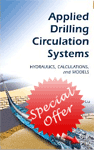Drilling Hydraulic Books

Share the joyWorking in the oil field and loving to share knowledge.

### 2 Responses to Optimum Drilling Flow Rate Example Calculation

1.Saeed says:

Hi,
•DrillingFormulas.Com says: